# Thread: Finding the Integral

1. ## Finding the Integral

$\displaystyle \int \sqrt{6-6x^2} dx$

I cant simplify it.
i got $\displaystyle sin\theta = \frac{\sqrt{6}}{6} x$
$\displaystyle cos\theta = \frac{\sqrt{6-6x^2}}{6}$

2. Hard to see where you might be at here. Just in case a picture helps...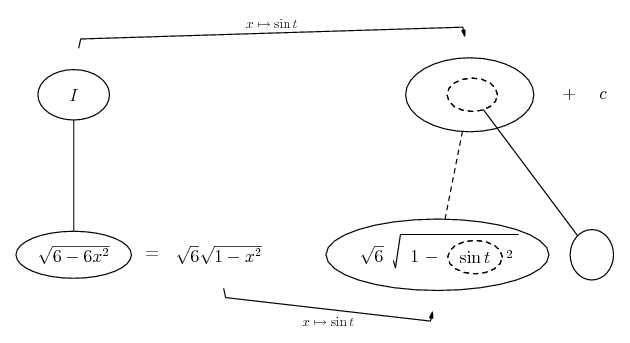... (key in spoiler)...

Spoiler:... is the chain rule. Straight continuous lines differentiate downwards (integrate up) with respect to the main present variable (in this case t), and the straight dashed line similarly but with respect to the dashed balloon expression (the inner function of the composite which is subject to the chain rule).

The general drift is...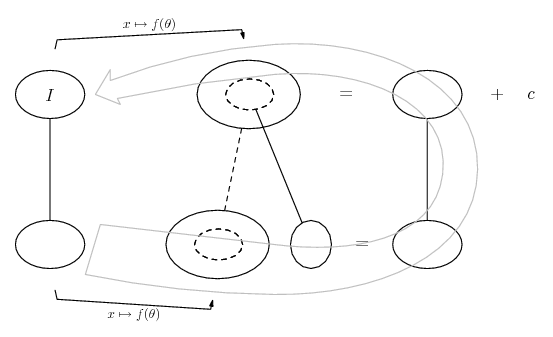The point is to see that we're going to integrate the original function as though it were the 'outer' derivative in a chain rule differentiation.

So next we fill out a bit more of the chain rule shape, to see the effect of having to multiply by the derivative of the inner function. Then we'll be able to fill the top of the shape by integrating the whole of the bottom level with respect to t...

Spoiler: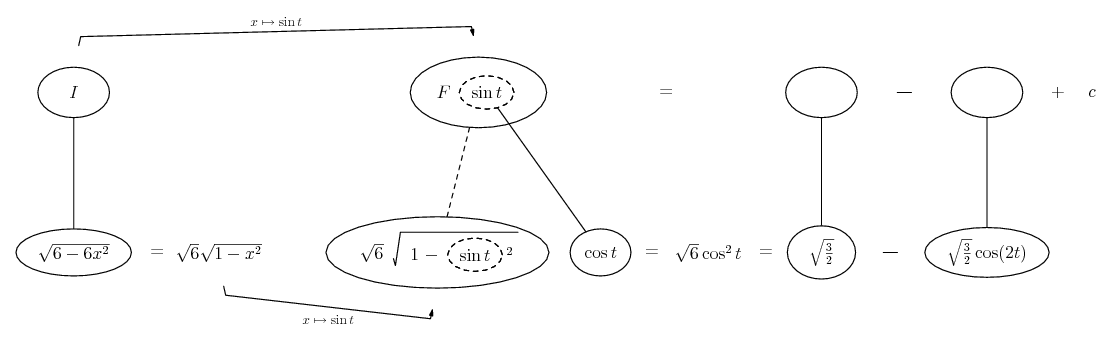Spoiler: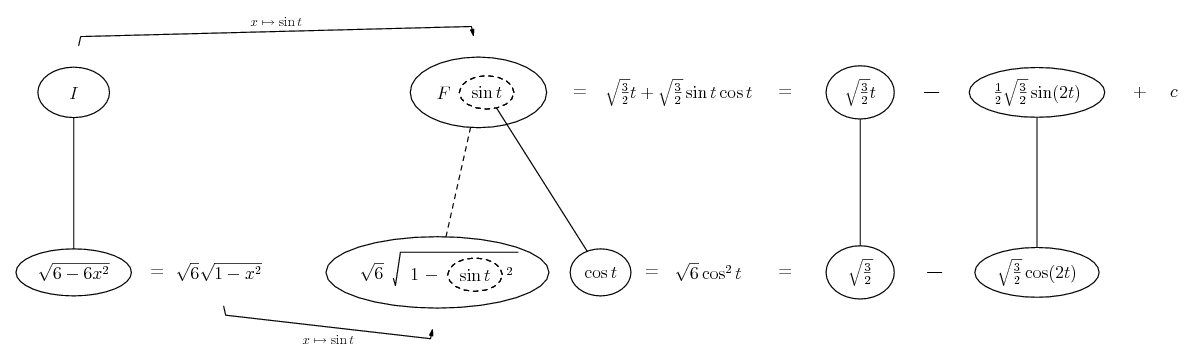Spoiler: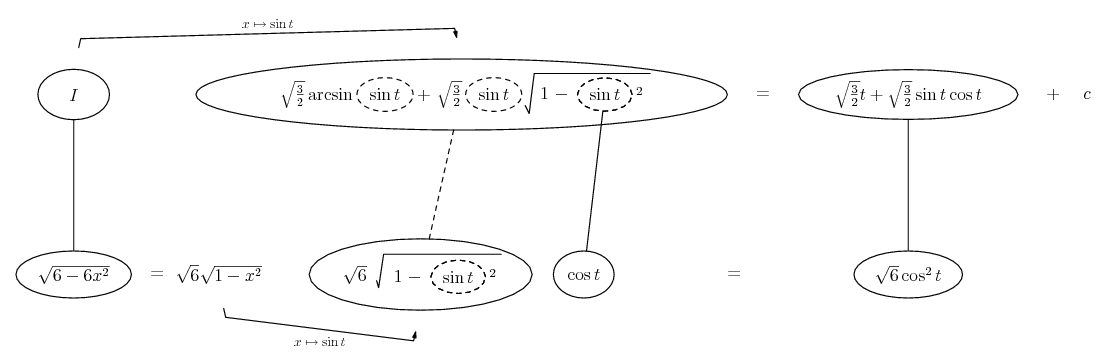Now it's clear enough how to write I in terms of x (writing x in place of each dashed ballon). Would show this, for completeness, but the pictures are being automatically reduced at the moment, so it would shrink out of sight if I did. Anyway, hope this helps!

_________________________________________

Don't integrate - balloontegrate!

Balloon Calculus; standard integrals, derivatives and methods

Balloon Calculus Drawing with LaTeX and Asymptote!

3. $\displaystyle \int{\sqrt{6 - 6x^2}\,dx} = \int{\sqrt{6(1-x^2)}\,dx}$

$\displaystyle = \int{\sqrt{6}\sqrt{1-x^2}\,dx}$

Now let $\displaystyle x = \sin{\theta}$ so that $\displaystyle dx= \cos{\theta}\,d\theta$ and the integral becomes

$\displaystyle \int{\sqrt{6}\sqrt{1 - \sin^2{\theta}}\,\cos{\theta}\,d\theta}$

$\displaystyle = \int{\sqrt{6}\sqrt{\cos^2{\theta}}\,\cos{\theta}\, d\theta}$

$\displaystyle = \int{\sqrt{6}\cos^2{\theta}\,d\theta}$

$\displaystyle = \int{\sqrt{6}\left(\frac{1}{2} + \frac{1}{2}\cos{2\theta}\right)\,d\theta}$

$\displaystyle = \frac{\sqrt{6}\theta}{2} + \frac{\sqrt{6}\sin{2\theta}}{4} + C$

$\displaystyle = \frac{\sqrt{6}\theta + \sqrt{6}\sin{\theta}\sqrt{1 - \sin^2{\theta}}}{2} + C$

$\displaystyle = \frac{\sqrt{6}\arcsin{x} + x\sqrt{6 - 6x^2}}{2} + C$.

4. Thank You that was really helpful couldnt get past that.

#### Search Tags

finding, integral